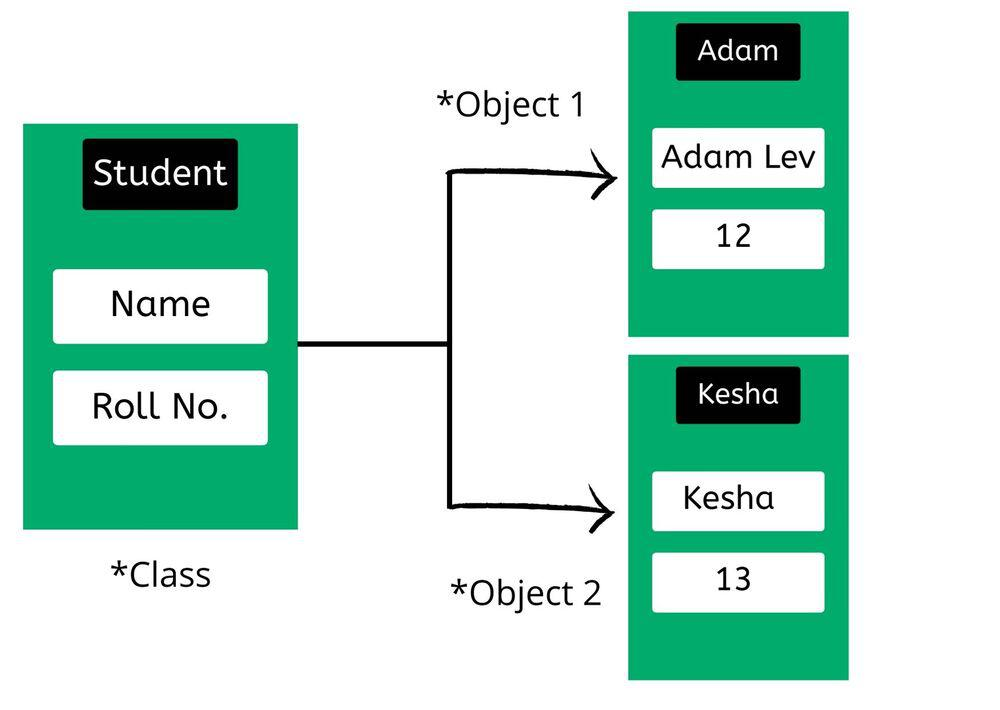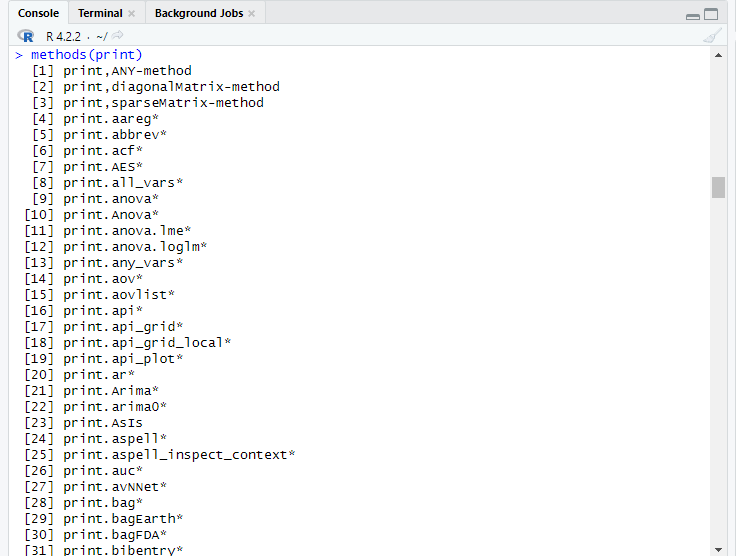# R – Object Oriented Programming

In this article, we will discuss Object-Oriented Programming (OOPs) in R Programming Language. We will discuss the S3 and S4 classes, the inheritance in these classes, and the methods provided by these classes.

## OOPs in R Programming Language:

In R programming, OOPs in R  provide classes and objects as its key tools to reduce and manage the complexity of the program. R is a functional language that uses concepts of OOPs. We can think of a class as a sketch of a car. It contains all the details about the model_name, model_no, engine, etc. Based on these descriptions we select a car. The car is the object. Each car object has its own characteristics and features. An object is also called an instance of a class and the process of creating this object is called instantiation. In R S3 and S4 classes are the two most important classes for object-oriented programming. But before going discussing these classes let’s see a brief about classes and objects.

## Class and Object

Class is the blueprint or a prototype from which objects are made by encapsulating data members and functions. An object is a data structure that contains some methods that act upon its attributes.R – Object Oriented Programming

## S3 Class

S3 class does not have a predefined definition and is able to dispatch. In this class, the generic function makes a call to the method. Easy implementation of S3 is possible because it differs from the traditional programming language Java, C++, and C# which implements Object Oriented message passing.

### Creating S3 Class

To create an object of this class we will create a list that will contain all the class members. Then this list is passed to the class() method as an argument.

Syntax:

variable_name <- list(attribute1, attribute2, attribute3….attributeN)

Example:

In the following code, a Student class is defined. An appropriate class name is given having attributes student’s name and roll number. Then the object of the student class is created and invoked.

## R

 `# List creation with its attributes name` `# and roll no.` `a <- ``list``(name=``"Adam"``, Roll_No=15)`   `# Defining a class "Student"` `class``(a) <- ``"Student"`   `# Creation of object` `a`

Output:

```\$name

\$Roll_No
 15

attr(, "class")
 "Student"```

### Generic Functions

The generic functions are a good example of polymorphism. To understand the concept of generic functions consider the print() function. The print() function is a collection of various print functions that are created for different data types and data structures in the R Programming Language. It calls the appropriate function depending on the type of object passed as an argument. We can see the various implementation of print functions using the methods() function.

Example: Seeing different types of the print function

## R

 `methods``(print)`

Output:Print methods

Now let’s create a generic function of our own. We will create the print function for our class that will print all the members in our specified format. But before creating a print function let’s create what the print function does to our class.

Example:

## R

 `# List creation with its attributes name` `# and roll no.` `a = ``list``(name=``"Adam"``, Roll_No=15)`   `# Defining a class "Student"` `class``(a) = ``"Student"`   `# Creation of object` `print``(a)`

Output:

```\$name

\$Roll_No
 15

attr(,"class")
 "Student"```

Now let’s print all the members in our specified format. Consider the below example –

Example:

## R

 `print.Student <- ``function``(obj){` `  ``cat``(``"name: "` `,obj\$name, ``"\n"``)` `  ``cat``(``"Roll No: "``, obj\$Roll_No, ``"\n"``)` `}`   `print``(a)`

Output:

```name:  Adam
Roll No:  15 ```

### Attributes

Attributes of an object do not affect the value of an object, but they are a piece of extra information that is used to handle the objects. The function attributes() can be used to view the attributes of an object.

Examples: An S3 object is created and its attributes are displayed.

## R

 `attributes``(a)`

Output:

```\$names
'name''Roll_No'
\$class
'Student'```

Also, you can add attributes to an object by using attr.

## R

 `attr``(a, ``"age"``)<-``c``(18)` `attributes``(a)`

Output:

```\$names
'name''Roll_No'
\$class
'Student'
\$age
18```

### Inheritance in S3 Class

Inheritance is an important concept in OOP(object-oriented programming) which allows one class to derive the features and functionalities of another class. This feature facilitates code-reusability.

S3 class in R programming language has no formal and fixed definition. In an S3 object, a list with its class attribute is set to a class name. S3 class objects inherit only methods from their base class.

Example:

In the following code, inheritance is done using S3 class, firstly the object is created of the class student.

## R

 `# student function with argument ` `# name(n) and roll_no(r)` `student <- ``function``(n, r) {` `    ``value <- ``list``(name=n, Roll=r)` `    ``attr``(value, ``"class"``) < - ``"student"` `    ``value` `}`

Then, the method is defined to print the details of the student.

## R

 `# 'print.student' method created` `print.student <- ``function``(obj) {`   `    ``# 'cat' function is used to concatenate` `    ``# strings` `    ``cat``(``"Name:"``, obj\$name, ``"\n"``)` `    ``cat``(``"Roll"``, obj\$roll, ``"\n"``)}`

Now, inheritance is done while creating another class by doing class(obj) <- c(child, parent).

## R

 `s <- ``list``(name=``"Kesha"``, Roll=21, country=``"India"``)`   `# child class 'Student' inherits` `# parent class 'student'` `class``(s) <- ``c``(``"Student"``, ``"student"``)` `s`

Output:

```Name: Kesha
Roll: 21 ```

The following code overwrites the method for class students.

## R

 `# 'Student' class object is passed` `# in the function of class 'student'`   `print.student <- ``function``(obj) {` `    ``cat``(obj\$name, ``"is from"``, obj\$country, ``"\n"``)` `}` `s`

Output:

`Kesha is from India`

## S4 Class

S4 class has a predefined definition. It contains functions for defining methods and generics. It makes multiple dispatches easy. This class contains auxiliary functions for defining methods and generics.

### Creating S4 class and object

setClass() command is used to create S4 class. Following is the syntax for setclass command which denotes myclass with slots containing name and rollno.

Syntax:

setClass(“myclass”, slots=list(name=”character”, Roll_No=”numeric”))

The new() function is used to create an object of the S4 class. In this function, we will pass the class name as well as the value for the slots.

Example:

## R

 `# Function setClass() command used` `# to create S4 class containing list of slots.` `setClass``(``"Student"``, slots=``list``(name=``"character"``,` `                               ``Roll_No=``"numeric"``))`   `# 'new' keyword used to create object of ` `# class 'Student'` `a <- ``new``(``"Student"``, name=``"Adam"``, Roll_No=20)`   `# Calling object` `a`

Output:

```Slot "name":

Slot "Roll_No":
 20```

### Create S4 objects from the generator function

setClass() returns a generator function that helps in creating objects and it acts as a constructor.

Example:

## R

 `stud <- ``setClass``(``"Student"``, slots=``list``(name=``"character"``,` `                               ``Roll_No=``"numeric"``))`   `# Calling object` `stud`

Output:

```class generator function for class “Student” from package ‘.GlobalEnv’
function (...)
new("Student", ...)```

Now the above-created stud function will act as the constructor for the Student class. It will behave as the new() function.

Example:

## R

 `stud``(name=``"Adam"``, Roll_No=15)`

Output:

```An object of class "Student"
Slot "name":

Slot "Roll_No":
 15```

### Inheritance in S4 class

S4 class in R programming have proper definition and derived classes will be able to inherit both attributes and methods from its base class. For this, we will first create a base class with appropriate slots and will create a generic function for that class. Then we will create a derived class that will inherit using the contains parameter. The derived class will inherit the members as well as functions from the base class.

Example:

## R

 `# Define S4 class` `setClass``(``"student"``,` `         ``slots=``list``(name=``"character"``,` `                    ``age=``"numeric"``, rno=``"numeric"``)` `         ``)`   `# Defining a function to display object details` `setMethod``(``"show"``, ``"student"``,` `          ``function``(obj){` `              ``cat``(obj@name, ``"\n"``)` `              ``cat``(obj@age, ``"\n"``)` `              ``cat``(obj@rno, ``"\n"``)` `          ``}` `          ``)`   `# Inherit from student` `setClass``(``"InternationalStudent"``,` `         ``slots=``list``(country=``"character"``),` `         ``contains=``"student"` `         ``)`   `# Rest of the attributes will be inherited from student` `s <- ``new``(``"InternationalStudent"``, name=``"Adam"``,` `          ``age=22, rno=15, country=``"India"``)` `show``(s)`

Output:

```Adam
22
15```

The reasons for defining both S3 and S4 classes are as follows:

• S4 class alone will not be seen if the S3 generic function is called directly. This will be the case, for example, if some function calls unique() from a package that does not make that function an S4 generic.
• However, primitive functions and operators are exceptions: The internal C code will look for S4 methods if and only if the object is an S4 object. S4 method dispatch would be used to dispatch any binary operator calls where either of the operands was an S4 object.
• S3 class alone will not be called if there is any eligible non-default S4 method.

So if a package defined an S3 method for unique for an S4 class but another package defined an S4 method for a superclass of that class, the superclass method would be chosen, probably not what was intended.

Whether you're preparing for your first job interview or aiming to upskill in this ever-evolving tech landscape, GeeksforGeeks Courses are your key to success. We provide top-quality content at affordable prices, all geared towards accelerating your growth in a time-bound manner. Join the millions we've already empowered, and we're here to do the same for you. Don't miss out - check it out now!

Previous
Next